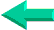### This is an excerpt from Pierre Leclerc (www.excel-vba.com)

 What can be done with SUMIFCan be done more easily with SUMPRODUCTWhat can't be done with SUMIFCan be done with SUMPRODUCT

# The SUMIF Function

 Summing with Excel's SUMIF Function Let's say that you have a set of data with columns for dates, products, cities, salespersons, quantities and unit prices. With the SUMIF function, you can sum quantities based on a single criteria like date OR product OR salesperson... SUMPRODUCT is like Excel SUMIF on steroids, with SUMPRODUCT you can sum quantities based on date AND product AND salesperson AND any number of other criteria... When you use Excel SUMPRODUCT function in a report to look at a set of data, you can just change the data and the report is AUTOMATICALLY refreshed.

The Excel SUMIF Function

The general format for the SUMIF formula is as follow:
=SUMIF(range where criteria applies, criteria, sum what)
Applied to the following table, the result of the formula

=SUMIF(C2:C7,"=John",D2:D7)
the result should be "14":

 A B C D 1 Month State Name Amount 2 Ma MI John 2 3 Ju NY Peter 6 4 Ma PA John 8 5 De NH Peter 3 6 No RI John 4 7 Oc FL Peter 6

The Excel SUMIF function is limited to one criteria (one column). If you want to sum the amounts based on month, State and name, discover the very simple SUMPRODUCT function with which you can use 2, 3 or more criteria with "or"  "and"...SUMPRODUCT is like SUMIF on steroids.Back home